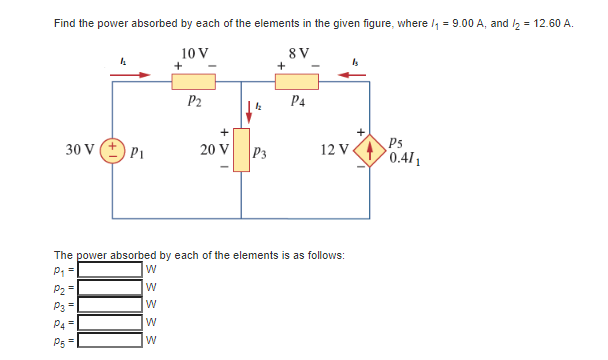# Question Solved1 AnswerFind the power absorbed by each of the elements in the given figure, where I1= 9 A and I2 = 12.60 A Find the power absorbed by each of the elements in the given figure, where I7 = 9.00 A, and 12 = 12.60 A. 10 V 8 V 1 + + P2 P4 12 + 30 V P1 20 V 12 v 411 P3 222 The power absorbed by each of the elements is as follows: p1 w W P3 W P4= W P5 wZK54U2 The Asker · Electrical Engineering

Find the power absorbed by each of the elements in the given figure, where I1= 9 A and I2 = 12.60 ATranscribed Image Text: Find the power absorbed by each of the elements in the given figure, where I7 = 9.00 A, and 12 = 12.60 A. 10 V 8 V 1 + + P2 P4 12 + 30 V P1 20 V 12 v 411 P3 222 The power absorbed by each of the elements is as follows: p1 w W P3 W P4= W P5 w
More
Transcribed Image Text: Find the power absorbed by each of the elements in the given figure, where I7 = 9.00 A, and 12 = 12.60 A. 10 V 8 V 1 + + P2 P4 12 + 30 V P1 20 V 12 v 411 P3 222 The power absorbed by each of the elements is as follows: p1 w W P3 W P4= W P5 w